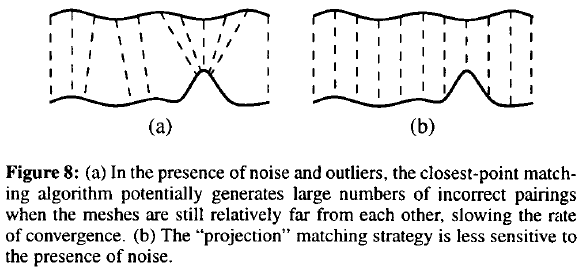# Efficient ICP Variants

##### Posted on Nov 07, 2020 (Update: Nov 08, 2020)

Many variants of ICP have been proposed, affecting all phases of the algorithm from the selection and matching of points to the minimization strategy. The paper evaluate their effects on the speed with which the correct alignment is reached.

## Introduction: Taxonomy of ICP variants

The paper

• assumes that a rough initial alignment is always available
• focuses only on aligning a single pair of meshes, and do not address the global registration problem

ICP is introduced by Chen and Medioni (1991) and Besl and McKay (1992).

The paper classify the variants as affecting one of six stages of the algorithm:

• selection of some set of points in one or both meshes
• matching these points to samples in the other mesh
• weighting the corresponding pairs appropriately
• rejecting certain pairs based on looking at each pair individually or considering the entire set of pairs.
• assigning an error metric based on the point sets.
• minimizing the error metric.

## Comparison Methodology

Goal: compare the convergence characteristic of several ICP variants

Choose a baseline combination of variants, and examining performance as individual ICP stages are varied. The selected baseline is Pulli (1999), incorporating the following features:

• random sampling of points on both meshes
• matching each selected point to the closest sample in the other mesh that has a normal within 45 degrees of the source normal
• uniform (constant) weighting of point pairs
• rejection of pairs containing edge vertices, as well as a percentage of pairs with the largest point-to-point distances
• point-to-plane error metric
• the classic “select-match-minimize” iteration, rather than some other search for the alignment transform.

## Comparisons of ICP variants

### Selection of Points

• all available points
• uniform subsampling
• random sampling
• select source points from both meshes: slightly better than others when using “asymmetric” matching algorithm
• normal-space sampling: outperforms others in the “incised plane” scene

### Matching points

• closest point
• closest compatible point (normals within 45 degrees)
• normal shooting: find the intersection of the ray originating at the source point in the direction of the source point’s normal with the destination surface
• normal shooting to a compatible point (normals within 45 degrees)
• projection (reverse calibration): project the source point onto the destination mesh, from the point of view of the destination mesh’s range camera (HOW??)
• projection by search: project the source point onto the destination mesh, then perform a search in the destination range image. The search might use a metric based on point-to-point distance, point-to-ray distance, or compatibility of intensity.the closest-point algorithms are more sensitive to noise and tend to generate larger numbers of incorrect pairings than the other algorithms

But the closest-point algorithms might not have the fastest convergence rate for “easy” scenes, they are the most robust for “difficult” geometry.

the projection algorithm (TODO: check the details) does not offer the best convergence per iteration, each iteration is faster than an iteration of closest point finding or normal shooting because it is performed in constant time, rather than involving a closet-point search.

### Weighting of Pairs

• constant weight
• lower weights to pairs with greater point-to-point distances: $\text{weight} = 1-\frac{\text{Dist}(p_1,p_2)}{\text{Dist}_\max}$
• based on compatibility of normals
• based on the expected effect of scanner noise on the uncertainty in the error metric

In general, the effect of weighting on convergence rate will be small and highly data-dependent, and that the choice of a weighting function should be based on other factors.

### Rejecting Pairs

• a given (user-specified) distance apart
• the worst $n\%$ of pairs
• the distance is larger than some multiple of the standard deviation of distances
• not consistent with neighboring pairs
• on mesh boundaries

outlier rejection, though it may have effects on the accuracy and stability with which the correct alignment is determined, in general does not improve the speed of convergence.

### Error Metric and Minimization

error metrics:

• sum of squared distances between corresponding points
• sum of squared distances from each source point to the plane containing the destination point and oriented perpendicular to the destination normal

several ways to search:

• “classic” ICP without extrapolation
• “classic” ICP with extrapolation